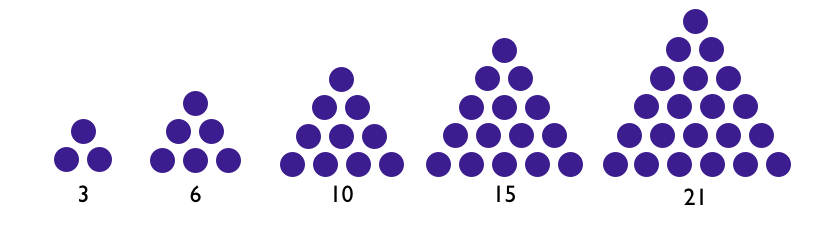# Triangle Numbers

Algebra Level 3To construct the $n$th triangle number, start with a row of $n$ dots. Then add a row with $n-1$ dots. Continue, adding a row with one fewer dot in every step, until a row with one dot is added. The drawing above show the second through sixth triangle numbers: $T_2 = 3, T_3 = 6, T_4 = 10, T_5 = 15, T_6 = 21$.

How much is $T_{99} + T_{100}$?

Try to answer without a calculator.